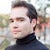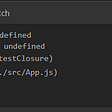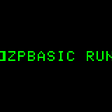# CoDEVIANT #20 (10/7/20)-Strings and Things (Soup is good food)

`function isPalindrome(string) { if(string.length === 1) {  return true } string.split('') let left = 0 let right = string.length - 1 while(left < string.length && left !== right) {  if(string[left] == string[right]) {   left++   right--  } else {   return false  } } return true}`
`if(string.length === 1) { return true}`
`string.split('')`
`let left = 0let right = string.length - 1`
• We want left to not be longer than the array itself, otherwise that would be silly and we’d go on to infinity and your computer would crash.
• We want left not equaling right because if it does, then they are pointing at the same element in the array which would mean they are at the one unique value in the potential palindrome
`while(left < string.length && left !== right) {   ...}`
`while(left < string.length && left !== right) {   if(string[left] == string[right]){      left++      right--   } else {     return false   }}`
`return true`
`function isPalindrome(string) { if(string.length === 1) {  return true } string.split('') let left = 0 let right = string.length - 1 while(left < string.length && left !== right) {  if(string[left] == string[right]) {   left++   right--  } else {   return false  } } return true}`
`function isPalindrome(string) {  let reversedString = ''  for(let i = string.length - 1; i >=0; i--){     reversedString += string[i]  }  return string === reversedString}`
`let reversedString = ''`
• makes the iterator (i) equal the length of the string minus one
• iterates over the length of the string
• stays active as long as the iterator (i) is more than or equal to 0
• in each loop decrements the iterator (i)
`for(let i = string.length - 1; i >= 0; i--) {   ...}`
`for(let i = string.length - 1; i >= 0; i--) {   reversedString += string[i]}`
`return string === reversedString`
`function caesarCipherEncryptor(string, key) { let alphabet = ['a','b','c','d','e','f','g',         'h','i','j','k','l','m','n',         'o','p','q','r','s','t','u',         'v','w','x','y','z'] let arr = [] string = string.split('') string.forEach(el => {  alphabet.forEach((letter, index) => {   if(el === letter) {    let num = index + key    while(num > 25 ) {     num = Math.abs(alphabet.length - num)    }    arr.push(num)   }  }) }) return arr.map(el => {  return alphabet[el] }).join('')}`
`let alphabet = ['a','b','c','d','e','f','g',         'h','i','j','k','l','m','n',         'o','p','q','r','s','t','u',         'v','w','x','y','z']`
`let arr = []`
`string = string.split('')`
`string.forEach(el => {   ...})`
`string.forEach(el => {   alphabet.forEach((letter, index) => {      ...   })})`
`string.forEach(el => {   alphabet.forEach((letter, index) => {      if(el === letter) {         let num = index + key      }   })})`
`string.forEach(el => {   alphabet.forEach((letter, index) => {      if(el === letter) {         let num = index + key      }      while(num > 25) {         num = Math.abs(alphabet.length - num)      }      arr.push(num)   })})`
`return arr.map(el => {   return alphabet[el]}).join('')`
`function caesarCipherEncryptor(string, key) { let newLetters = [] let newKey = key % 26 for(const letter of string) {  newLetters.push(getNewLetter(letter, newKey)) } return newLetters.join('')  function getNewLetter(letter, key) {  const newLetterCode = letter.charCodeAt() + key  return newLetterCode <= 122    ? String.fromCharCode(newLetterCode)   : String.fromCharCode(96 + (newLetterCode % 122)) }}`
`let newLetters = []`
`let newKey = key % 26`
• *This is a way of iterating over each character in a string without first turning it into an array. I wouldn’t have thought of it and it’s not something I’ve seen alot, but it’s cool to know about**
`for(const letter of string) {   newLetters.push(getNewLetter(letter, newKey))}`
• a in unicode is 97
• z in unicode is 122
`function getNewLetter(letter, key) {  const newLetterCode = letter.charCodeAt() + key}`
• If newLetterCode is 122 or under, we return the result of String.fromCharCode(newLetterCode)
• If newLetterCode is over 122, we return the result of String.fromCharCode(96 + (newLetterCode % 122))
`function getNewLetter(letter, key) {  const newLetterCode = letter.charCodeAt() + key  return newLetterCode <= 122     ? String.fromCharCode(newLetterCode)     : String.fromCharCode(96 + (newLetterCode & 122))}`
`return newLetters.join('')`
`function caesarCipherEncryptor(string, key) { let newLetters = [] let newKey = key % 26 for(const letter of string) {  newLetters.push(getNewLetter(letter, newKey)) } return newLetters.join('')  function getNewLetter(letter, key) {  const newLetterCode = letter.charCodeAt() + key  return newLetterCode <= 122    ? String.fromCharCode(newLetterCode)   : String.fromCharCode(96 + (newLetterCode % 122)) }}`

--

--

--

is a web developer, opera singer, actor, and lover of cats. (adrian-rosales.tech)

Love podcasts or audiobooks? Learn on the go with our new app.

## Bootstrapping a Typescript Worker## How to Create a Simple Web Server using Node.js## NestJs: Firebase Auth secured NestJs resource server## “Super” and “Extends” In JavaScript ES6 - Understanding The Tough Parts## The Complete Guide to Forms in React## Creating a RESTful Web API with Node.js and Express.js from scratch## How To Build A Blog with WordPress and Gatsby.js — Part 1## When to use useEffect in React?is a web developer, opera singer, actor, and lover of cats. (adrian-rosales.tech)

## Listen Up## Use Chrome devtools understanding Closure .## BASIC Necessities